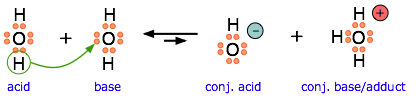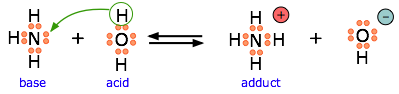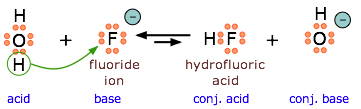# 17.11: Lewis Acids and Bases

$$\newcommand{\vecs}{\overset { \rightharpoonup} {\mathbf{#1}} }$$ $$\newcommand{\vecd}{\overset{-\!-\!\rightharpoonup}{\vphantom{a}\smash {#1}}}$$$$\newcommand{\id}{\mathrm{id}}$$ $$\newcommand{\Span}{\mathrm{span}}$$ $$\newcommand{\kernel}{\mathrm{null}\,}$$ $$\newcommand{\range}{\mathrm{range}\,}$$ $$\newcommand{\RealPart}{\mathrm{Re}}$$ $$\newcommand{\ImaginaryPart}{\mathrm{Im}}$$ $$\newcommand{\Argument}{\mathrm{Arg}}$$ $$\newcommand{\norm}{\| #1 \|}$$ $$\newcommand{\inner}{\langle #1, #2 \rangle}$$ $$\newcommand{\Span}{\mathrm{span}}$$ $$\newcommand{\id}{\mathrm{id}}$$ $$\newcommand{\Span}{\mathrm{span}}$$ $$\newcommand{\kernel}{\mathrm{null}\,}$$ $$\newcommand{\range}{\mathrm{range}\,}$$ $$\newcommand{\RealPart}{\mathrm{Re}}$$ $$\newcommand{\ImaginaryPart}{\mathrm{Im}}$$ $$\newcommand{\Argument}{\mathrm{Arg}}$$ $$\newcommand{\norm}{\| #1 \|}$$ $$\newcommand{\inner}{\langle #1, #2 \rangle}$$ $$\newcommand{\Span}{\mathrm{span}}$$$$\newcommand{\AA}{\unicode[.8,0]{x212B}}$$

##### Learning Objectives

Make sure you thoroughly understand the following essential ideas which have been presented above. It is especially important that you know the precise meanings of all the highlighted terms in the context of this topic.

• Write the equation for the proton transfer reaction involving a Brønsted-Lowry acid or base, and show how it can be interpreted as an electron-pair transfer reaction, clearly identifying the donor and acceptor.
• Give an example of a Lewis acid-base reaction that does not involve protons.
• Write equations illustrating the behavior of a given non-aqueous acid-base system.

The Brønsted-Lowry proton donor-acceptor concept has been one of the most successful theories of Chemistry. But as with any such theory, it is fair to ask if this is not just a special case of a more general theory that could encompass an even broader range of chemical science. In 1916, G.N. Lewis of the University of California proposed that the electron pair is the dominant actor in acid-base chemistry. The Lewis theory did not become very well known until about 1923 (the same year that Brønsted and Lowry published their work), but since then it has been recognized as a very powerful tool for describing chemical reactions of widely different kinds and is widely used in organic and inorganic chemistry. According to Lewis,

• An acid is a substance that accepts a pair of electrons, and in doing so, forms a covalent bond with the entity that supplies the electrons.
• A base is a substance that donates an unshared pair of electrons to a recipient species with which the electrons can be shared.

In modern chemistry, electron donors are often referred to as nucleophiles, while acceptors are electrophiles.

## Proton-Transfer Reactions Involve Electron-Pair Transfer

Just as any Arrhenius acid is also a Brønsted acid, any Brønsted acid is also a Lewis acid, so the various acid-base concepts are all "upward compatible". Although we do not really need to think about electron-pair transfers when we deal with ordinary aqueous-solution acid-base reactions, it is important to understand that it is the opportunity for electron-pair sharing that enables proton transfer to take place.This equation for a simple acid-base neutralization shows how the Brønsted and Lewis definitions are really just different views of the same process. Take special note of the following points:

• The arrow shows the movement of a proton from the hydronium ion to the hydroxide ion.
• Note carefully that the electron-pairs themselves do not move; they remain attached to their central atoms. The electron pair on the base is "donated" to the acceptor (the proton) only in the sense that it ends up being shared with the acceptor, rather than being the exclusive property of the oxygen atom in the hydroxide ion.
• Although the hydronium ion is the nominal Lewis acid here, it does not itself accept an electron pair, but acts merely as the source of the proton that coordinates with the Lewis base.

The point about the electron-pair remaining on the donor species is especially important to bear in mind. For one thing, it distinguishes a Lewis acid-base reaction from an oxidation-reduction reaction, in which a physical transfer of one or more electrons from donor to acceptor does occur. The product of a Lewis acid-base reaction is known formally as an "adduct" or "complex", although we do not ordinarily use these terms for simple proton-transfer reactions such as the one in the above example. Here, the proton combines with the hydroxide ion to form the "adduct" H2O. The following examples illustrate these points for some other proton-transfer reactions that you should already be familiar with.Another example, showing the autoprotolysis of water. Note that the conjugate base is also the adduct.Ammonia is both a Brønsted and a Lewis base, owing to the unshared electron pair on the nitrogen. The reverse of this reaction represents the hydrolysis of the ammonium ion.Because $$\ce{HF}$$ is a weak acid, fluoride salts behave as bases in aqueous solution. As a Lewis base, F accepts a proton from water, which is transformed into a hydroxide ion.The bisulfite ion is amphiprotic and can act as an electron donor or acceptor.

## Acid-base Reactions without Transferring Protons

The major utility of the Lewis definition is that it extends the concept of acids and bases beyond the realm of proton transfer reactions. The classic example is the reaction of boron trifluoride with ammonia to form an adduct:

$\ce{BF_3 + NH_3 \rightarrow F_3B-NH_3}$

One of the most commonly-encountered kinds of Lewis acid-base reactions occurs when electron-donating ligands form coordination complexes with transition-metal ions.Figure $$\PageIndex{1}$$: The tin atom in SnCl4 can expand its valence shell by utilizing a pair of d-orbitals, changing its hybridization from sp3 to sp3d2.
##### Exercise $$\PageIndex{1}$$

Here are several more examples of Lewis acid-base reactions that cannot be accommodated within the Brønsted or Arrhenius models. Identify the Lewis acid and Lewis base in each reaction.

1. $$\ce{Al(OH)_3 + OH^{–} \rightarrow Al(OH)_4^–}$$
2. $$\ce{SnS_2 + S^{2–} \rightarrow SnS_3^{2–}}$$
3. $$\ce{Cd(CN)_2 + 2 CN^– \rightarrow Cd(CN)_4^{2+}}$$
4. $$\ce{AgCl + 2 NH_3 \rightarrow Ag(NH_3)_2^+ + Cl^–}$$
5. $$\ce{Fe^{2+} + NO \rightarrow Fe(NO)^{2+}}$$
6. $$\ce{Ni^{2+} + 6 NH_3 \rightarrow Ni(NH_3)_5^{2+}}$$

Applications to organic reaction mechanisms

Although organic chemistry is beyond the scope of these lessons, it is instructive to see how electron donors and acceptors play a role in chemical reactions. The following two diagrams show the mechanisms of two common types of reactions initiated by simple inorganic Lewis acids:In each case, the species labeled "Complex" is an intermediate that decomposes into the products, which are conjugates of the original acid and base pairs. The electric charges indicated in the complexes are formal charges, but those in the products are "real".

In reaction 1, the incomplete octet of the aluminum atom in $$\ce{AlCl3}$$ serves as a better electron acceptor to the chlorine atom than does the isobutyl part of the base. In reaction 2, the pair of non-bonding electrons on the dimethyl ether coordinates with the electron-deficient boron atom, leading to a complex that breaks down by releasing a bromide ion.

## Non-aqueous Protonic Acid-Base Systems

We ordinarily think of Brønsted-Lowry acid-base reactions as taking place in aqueous solutions, but this need not always be the case. A more general view encompasses a variety of acid-base solvent systems, of which the water system is only one (Table $$\PageIndex{1}$$). Each of these has as its basis an amphiprotic solvent (one capable of undergoing autoprotolysis), in parallel with the familiar case of water.

The ammonia system is one of the most common non-aqueous system in Chemistry. Liquid ammonia boils at –33° C, and can conveniently be maintained as a liquid by cooling with dry ice (–77° C). It is a good solvent for substances that also dissolve in water, such as ionic salts and organic compounds since it is capable of forming hydrogen bonds. However, many other familiar substances can also serve as the basis of protonic solvent systems as Table $$\PageIndex{1}$$ indicates:

Table $$\PageIndex{1}$$: Popular Solvent systems
solvent
autoprotolysis reaction
pKap
water 2 H2O → H3O+ + OH 14
ammonia 2 NH3 → NH4+ + NH2 33
acetic acid 2 CH3COOH → CH3COOH2+ + CH3COO 13
ethanol 2 C2H5OH → C2H5OH2+ + C2H5O 19
hydrogen peroxide 2 HO-OH → HO-OH2+ + HO-O 13
hydrofluoric acid 2 HF → H2F+ + F 10
sulfuric acid 2 H2SO4 → H3SO4+ + HSO4 3.5

One use of nonaqueous acid-base systems is to examine the relative strengths of the strong acids and bases, whose strengths are "leveled" by the fact that they are all totally converted into H3O+ or OH ions in water. By studying them in appropriate non-aqueous solvents which are poorer acceptors or donors of protons, their relative strengths can be determined.Figure $$\PageIndex{2}$$: Use of non-aqueous solvents allows the study of strong acids that are hindered by the "leveling" of the solvent.

17.11: Lewis Acids and Bases is shared under a not declared license and was authored, remixed, and/or curated by LibreTexts.